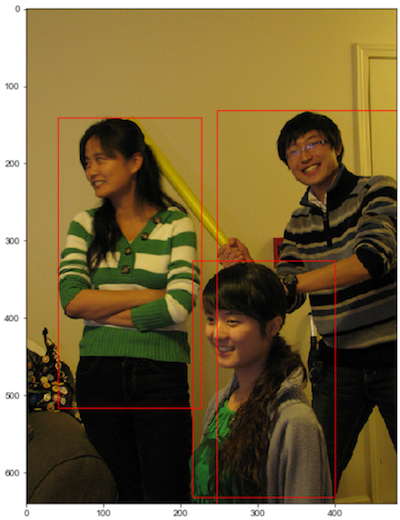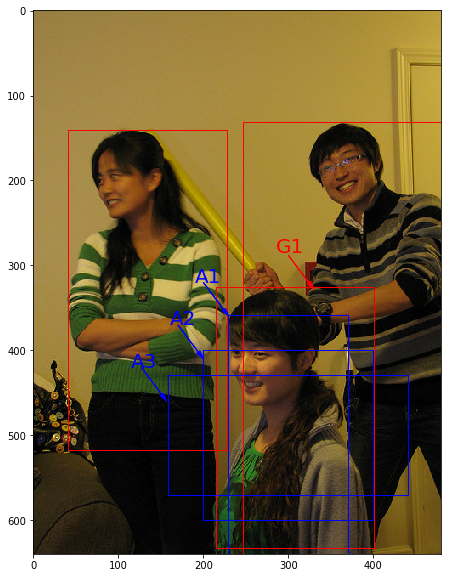# 边界框（bounding box）¶1. $$(x_1, y_1, x_2, y_2)$$，其中$$(x_1, y_1)$$是矩形框左上角的坐标，$$(x_2, y_2)$$是矩形框右下角的坐标。图1 中3个红色矩形框用$$xyxy$$格式表示如下：

• 左：$$(40.93, 141.1, 226.99, 515.73)$$

• 中：$$(214.29, 325.03, 399.82, 631.37)$$

• 右：$$(247.2, 131.62, 480.0, 639.32)$$

1. $$xywh$$，即$$(x, y, w, h)$$，其中$$(x, y)$$是矩形框中心点的坐标，$$w$$是矩形框的宽度，$$h$$是矩形框的高度。

1. 在阅读代码时，请注意使用的是哪一种格式的表示方式。

2. 图片坐标的原点在左上角，$$x$$轴向右为正方向，$$y$$轴向下为正方向。

# 锚框（Anchor box）¶

1. 人为经验选取

2. k-means聚类

3. 作为超参数进行学习# 画图展示如何绘制边界框和锚框
import numpy as np
import matplotlib.pyplot as plt
import matplotlib.patches as patches
import math

# 定义画矩形框的程序
def draw_rectangle(currentAxis, bbox, edgecolor = 'k', facecolor = 'y', fill=False, linestyle='-'):
# currentAxis，坐标轴，通过plt.gca()获取
# bbox，边界框，包含四个数值的list， [x1, y1, x2, y2]
# edgecolor，边框线条颜色
# facecolor，填充颜色
# fill, 是否填充
# linestype，边框线型

# patches.Rectangle(xy, width, height,linewidth,edgecolor,facecolor,fill, linestyle)
# xy:左下角坐标; width:矩形框的宽; height:矩形框的高; linewidth:线宽; edgecolor:边界颜色; facecolor:填充颜色; fill:是否填充; linestyle:线断类型
rect=patches.Rectangle((bbox, bbox), bbox-bbox+1, bbox-bbox+1, linewidth=1,
edgecolor=edgecolor,facecolor=facecolor,fill=fill, linestyle=linestyle)

plt.figure(figsize=(10, 10))
# 传入图片路径
filename = '/home/aistudio/work/images/section3/000000086956.jpg'
plt.imshow(im)

# 使用xyxy格式表示物体真实框
bbox1 = [214.29, 325.03, 399.82, 631.37]
bbox2 = [40.93, 141.1, 226.99, 515.73]
bbox3 = [247.2, 131.62, 480.0, 639.32]

currentAxis=plt.gca()
# 绘制3个真实框
draw_rectangle(currentAxis, bbox1, edgecolor='r')
draw_rectangle(currentAxis, bbox2, edgecolor='r')
draw_rectangle(currentAxis, bbox3,edgecolor='r')

# 绘制锚框
def draw_anchor_box(center, length, scales, ratios, img_height, img_width):
"""
以center为中心，产生一系列锚框
其中length指定了一个基准的长度
scales是包含多种尺寸比例的list
ratios是包含多种长宽比的list
img_height和img_width是图片的尺寸，生成的锚框范围不能超出图片尺寸之外
"""
bboxes = []
for scale in scales:
for ratio in ratios:
h = length*scale*math.sqrt(ratio)
w = length*scale/math.sqrt(ratio)
x1 = max(center - w/2., 0.)
y1 = max(center - h/2., 0.)
x2 = min(center + w/2. - 1.0, img_width - 1.0)
y2 = min(center + h/2. - 1.0, img_height - 1.0)
print(center, center, w, h)
bboxes.append([x1, y1, x2, y2])

for bbox in bboxes:
draw_rectangle(currentAxis, bbox, edgecolor = 'b')

img_height = im.shape
img_width = im.shape
# 绘制锚框
draw_anchor_box([300., 500.], 100., [2.0], [0.5, 1.0, 2.0], img_height, img_width)

################# 以下为添加上图中的文字说明和箭头###############################
plt.text(285, 285, 'G1', color='red', fontsize=20)
plt.arrow(300, 288, 30, 40, color='red', width=0.001, length_includes_head=True, \

plt.text(190, 320, 'A1', color='blue', fontsize=20)
plt.arrow(200, 320, 30, 40, color='blue', width=0.001, length_includes_head=True, \